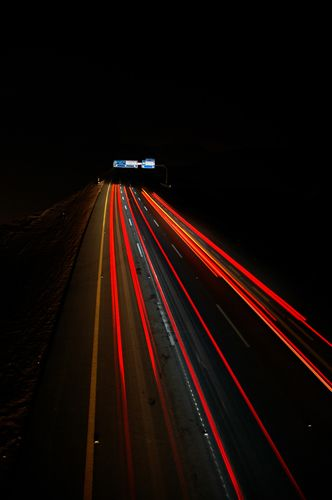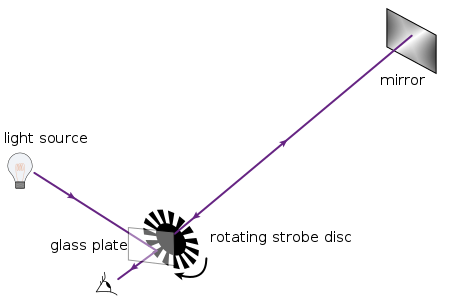# Measuring the Speed of Light

in #science4 years ago

We are told, in the physics class on the subject of special theory of relativity that
E=mc2.

That is Einstein's super famous mass-energy equivalence equation. An equation that was so popular, that it is the math equation of choice printing on many t-shirts, caps, etc.Light. By Yasser Alghofily [CC BY 2.0] , from Flickr Commons Licenced

In that equivalent energy equation
E= equivalent enerrgy measured in joules (J)
m= mass measured inkilograms (kg)
c= speed of light measured in meters per second (m/s or ms-1)

The c is a universal physical constant.
The c is derived from the Latin word celeritas which translates to swift. A great definition as the speed of light is light in a vacuum is 3 x 108 m/s or 300,000 km per second. Considering the circumference of the Earth is 40,075km, the speed of light goes around the earth up to 7 times in a second! That, I can agree, is swift.

The speed of light exact value is 299,792,458 meters per second in a vacuum, the approximate value of 300,000,000 meters per second is common as it simplifies mathematical calculations.

#### How did we get such a value?

299,792,457 meters per second or 186,000 miles per second is a massively small number. I have always wondered how they were able to measure it.

It is only natural we begin the story of the measurement from past attempts to measure light.

Again we begin the journey with the ancient Greeks; we owe some of the mathematical discovery to these ancient mathematicians.

Back then, it all started with some level of disagreement with a philosopher and a scientist in ancient Greece. The philosopher was Aristotle while the scientist was Empedocles. Aristotle was of the opinion that light does not travel. He proposes that light is instantaneous and does not travel. Empedocles, on the other hand, says that light travels and have a finite speed. A proposal that Aristotle opposed.

Galileo, the Italian astronomer, put up an experiment to determine the speed of light. It involves two experimenters, A and B, standing some distance away from one another with a special lantern that has an open and close shutter. B will open the lamp shutter on seeing A's lamp's light. This will allow A to measure the time lapse between when B observes the light and when A opened it. But as we mentioned earlier, the speed of light is very fast, so if the time difference is to be noticed more clearly, Galileo reasons that the experimenters should stand about 10 miles apart and view each other lanterns with a telescope.

The result of the experiment was published in the Galileo's book Discorsi e Dimostrazioni Matematiche Intorno a Due Nuove Scienze or Discourses and Mathematical Demonstrations Relating to Two New Sciences in 1638. Even though the method of measuring the speed of light using the two-lanterns-at-a- distance approach is erroneous, Galileo was able to infer from it that the speed of light finite and very fast.Ole Roemer's vector diagram depicting the eclipse of Jupiter's moon Io used to determine the speed of light. By Ole Roemer [CC0], from Wikimedia Commons Licenced

In 1676, thirty-eight years after this book, a Danish astronomer Ole Christensen Rømer made great scientific history by becoming the first person to make a quantitative measure of light's speed. The method employs the use of astronomy through the study of the eclipses that occur in the biggest planet of our solar system, between the Jupiter and the moons surrounding it, with how the observers on earth see the light from the eclipse at different times when observed in a different period of the year.

The diagram shows Jupiter (B) having an eclipse (DC)) with one of its four moons, Io. E, F, G, H, L and K shows the different locations in Earth's orbit as it moves around the Sun, A.

Through repeated logging of eclipses over a period of 8 years, Romer observed a pattern of a faster speed up rate of 3 1⁄2 minutes (per month) while orbiting Jupiter for the first six months which amounted to 22 minutes faster orbit time. Then in the next six months Io's orbitb of Jupiter slows down by same 3 1⁄2 minutes per month totaling the same 22 minutes of slow orbit time. He had a Eureka moment when he realised it was as a result of changing distance between Earth and Jupiter as both orbits the Sun.

The timing of the eclipses observed on Earth depends on the side of the Sun the Earth is orbiting on. The difference between the timing of the eclipse when the Earth is on the same side of the Sun as Jupiter and when it is on the opposite side of the Sun as Jupiter convinces Roemer that the light requires more time to get to the Earth. The light travels a farther distance to get to his telescope. This time is the speed of light.

Romer already knew the distance from the Sun to Earth is approximately 90 million miles which imply the diameter of the orbit is in the neighbourhood of 180 million miles.

Using the knowledge that it takes 22 minutes to cross the diameter of Earth's orbit plus the diameter of the earth, the Royal Academy in December 1676 was able to calculate the speed of light to be 2.14 x 10^8 m/s or about 130,000 miles per second or 210,000 kilometres per second.

The calculation is short of the modern calculated time of 299,792.458 kilometres per second by about 28%. This is not bad considering the method used more than 300 years ago.

Also using modern highly sophisticated telescopes, astronomers were able to calculate the number of minutes it takes light to cross the Earth's orbit from Jupiter is less than 22 minutes at 16 minutes and 40 seconds minutes.Fizeau's experimental setup with a notched wheel for determining the speed of light. By Fred the Oyster [CC BY-SA 4.0] , from Wikimedia Commons Licenced

Another scientist Armand Fizeau in 1849 made another good attempt to measure the speed of light using a source of light, mirror and a rotating wheel. A mirror placed 8km away reflects light through the wheel's teeth as it rotates. If the distance and rate of its spin of the wheel are known, we can then calculate the speed of light.

The method produced a more accurate measurement of the speed of light at 313,300 km/s or 194,700 miles per second. This result is just a mere 4.70% above the modern calculated value.

Other measurements were done by other scientist using various another method. Today, the speed of light, as measured in a vacuum, is accurate with the employment of helium-neon lasers combined with caesium or atomic clock known for high precision measurement to get the value of 299,792.458 km/s. That is the standard the general public, science student, teacher or researcher uses. But hey, someone almost got this 345 years ago by looking at Jupiter's eclipse.

REFERENCES

Sort:

The method by Romer in 1676 is by far my favourite method. To think that they did this ~400 years ago is just amazing.

I'm blown away by the thinking of all these ancient mathematicians, scientists, and engineers. Their thinking are certainly ahead of their times.